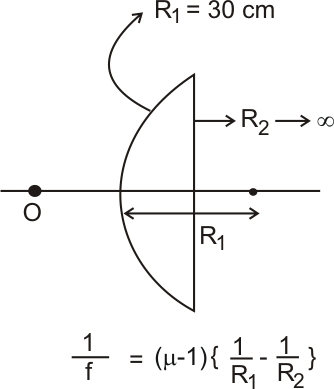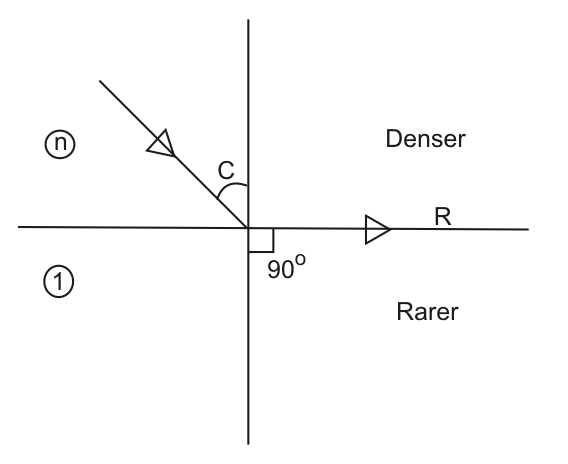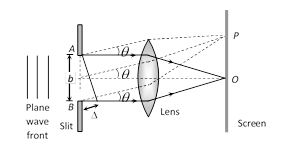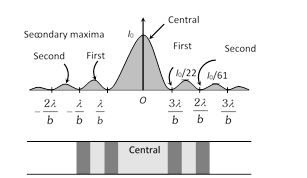Filter By

## All Questions

#### In an experiment for determination of refractive index of glass of a prism by, plot, it was found that a ray incident at angle 350, suffers a deviation of 400 and that it emerges at angle 790⋅  Ιn that case which of the following is closest to the maximum possible value of the refractive index ? Option: 1  1.5 Option: 2  1.6 Option: 3 1.7 Option: 4 1.8

This angle is greater than the 40° deviation angle already given, For greater μ, deviation will be even higher. Hence, μ of the given prism should be lesser than 1.5. Hence, the closest option will be 1.5.

#### A single slit of width b is illuminated by a coherent monochromatic light of wavelength λ. If the second and fourth minima in the diffraction pattern at a distance 1 m from the slit are at 3 cm and 6 cm respectively from the central maximum, what is the width of the central maximum ? (i.e. distance between first minimum on either side of the central maximum) Option: 1 1.5 cm Option: 2 3.0 cm Option: 3 4.5 cm Option: 4 6.0 cm

As the distance of nth minima from the central maximum is given as

Difference between second and fourth minimum =6−3=3

i.e

## Crack CUET with india's "Best Teachers"

• HD Video Lectures
• Unlimited Mock Tests
• Faculty Support#### A point object in air is in front of the curved surface of alens. The radius of curvature of the curved surface isand the refractive index of the lens material isthen the focal length of the lens (in cm) is _____________.    Option: 1 60 Option: 2 30 Option: 3 120 Option: 4 100So,So f= 60 cm

So the answer will be 60.

#### The magnifying power of a telescope with tube length  is . What is the focal length (in cm) of its eye piece?   Option: 1 30 Option: 2 40 Option: 3 10 Option: 4 20

So the correct answer is given in option 3.

## Crack NEET with "AI Coach"

• HD Video Lectures
• Unlimited Mock Tests
• Faculty Support#### The critical angle (in degree)of a medium for a specific wavelength, if the medium has relative permitivityand relative permeabilityfor this wavelength, will be :  Option: 1 15 Option: 2 30 Option: 3 45 Option: 4 60andBy using snells lawSo the correct option is 2.

#### In a young's double slit experiment 15 fringes are observed on a small portion of the screen when light of wavelength 500 nm is used. Ten fringes are observed on the same section of the screen when another light source of wavelengthis used. Then the value ofis ( in nm) _____. Option: 1 750 Option: 2 650 Option: 3 850 Option: 4 450## Crack JEE Main with "AI Coach"

• HD Video Lectures
• Unlimited Mock Tests
• Faculty Support#### Visible height of wavelengthcm falls normally on a single slit and produces a diffraction pattern. It is found that the second diffraction min. is at 60 from the central max. If the first minimum is produced at, theis close to,   Option: 1Option: 2Option: 3Option: 4Fraunhofer diffraction by a single slit -

Fraunhofer diffraction by a single slit

let's assume  a plane wave front is incident on a slit AB (of width b). Each and every part of the exposed part of the plane wave front (i.e. every part of the slit) acts as a source of secondary wavelets spreading in all directions. The diffraction is obtained on a screen placed at a large distance. (In practice, this condition is achieved by placing the screen at the focal plane of a converging lens placed just after the slit).•  The diffraction pattern consists of a central bright fringe (central maxima) surrounded by dark and bright lines (called secondary minima and maxima).
•  At point O on the screen, the central maxima is obtained. The wavelets originating from points A and B meets in the same phase at this point, hence at O, intensity is maximum

Secondary minima : For obtaining nth secondary minima at P on the screen, path difference between the diffracted waves1. Angular position of nth secondary minima:2. Distance of nth secondary minima from central maxima:where D = Distance between slit and screen.= Focal length of converging lens.

Secondary maxima : For nth secondary maxima at P on the screen.

Path difference; where n = 1, 2, 3 .....

(i) Angular position of nth secondary maxima(ii) Distance of nth secondary maxima from central maxima:Central maxima : The central maxima lies between the first minima on both sides.

(i) The Angular width d central maxima    =(ii) Linear width of central maximaIntensity distribution: if the intensity of the central maxima isthen the intensity of the first and secondary maxima are
found to be. Thus diffraction fringes are of unequal width and unequal intenstities.

(i) The mathematical expression for in intensity distribution on the screen is given by:whereis just a convenient connection between the anglethat locates a point on the viewing screening and light intensity I.Phase difference between the top and bottom ray from the slit width b.
Also(ii) As the slit width increases relative to wavelength the width of the control diffraction maxima decreases; that is, the light undergoes less flaring by the slit. The secondary maxima also decreases in width and becomes weaker.

(iii) If  b>>λ, the secondary maxima due to the slit disappear; we then no longer have single slit diffraction.

-So,For first minima,So option (3) is correct.

#### If we used a magnification of 375 from a compound microscope of tube length 150mm and an objective of focal length 5mm, the focal length of the eyepiece should be close to :    Option: 1 12mm  Option: 233mm Option: 3 22mm   Option: 4 2mm

The magnification is given by

So option (3) is correct.

## Crack CUET with india's "Best Teachers"

• HD Video Lectures
• Unlimited Mock Tests
• Faculty Support#### There is a small source of light at some depth below the surface of water (refractive index =) in a tank of large cross sectional surface area. Neglecting any reflection from the bottom and absorption by water, percentage of light that emerges out of surface is (nearly) : [ Use the fact that surface area of a spherical cap of height h and radius of curvature r is] Option: 1Option: 2Option: 3Option: 4Percentage of light =

Hence the correct option is (2).

#### In a double-slit experiment, at a certain point on the screen the path difference betweeen the two interfering waves isth of a wavelength. The ratio of the intensity of light at that point to that at the centre of a bright fringe is : Option: 1 0.568 Option: 2 0.853 Option: 3 0.760 Option: 4 0.672

Intensity is given byWhere IO=maximum intensity=intensity of light at the center of a bright fringe

and  using the Relationwe getHence the correct option is (2).